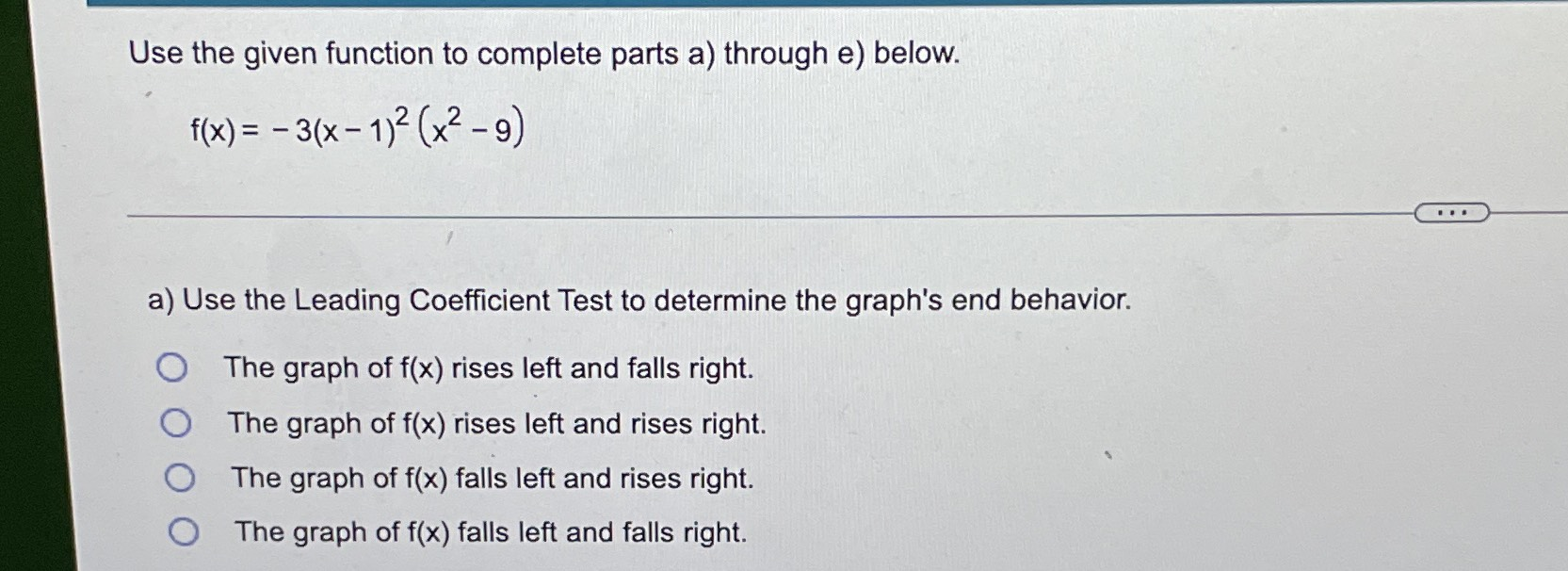### Still have math questions?

Algebra
QuestionUse the given function to complete parts a) through e) below. $$( x ) = - 3 ( x - 1 ) ^ { 2 } ( x ^ { 2 } - 9 )$$

a) Use the Leading Coefficient Test to determine the graph's end behavior.

The graph of $$f ( x )$$ rises left and falls right.

The graph of $$f ( x )$$ rises left and rises right.

The graph of $$f ( x )$$ falls left and rises right.

The graph of $$f ( x )$$ falls left and falls right.